# Higher-Order Functions

Higher-order functions are the pendant to First-Class Functions because higher-order functions can take functions as an argument or return them as a result.

## Higher-order functions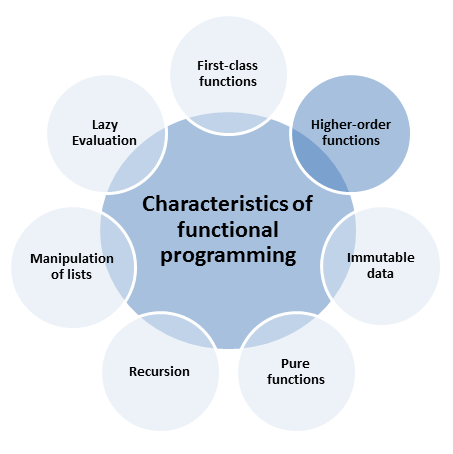### The three classics: map, filter, and fold

Each programming language supporting programming in the functional style supports at least three functions map, filter, and fold. map applies a function to each element of its list. filter removes all elements of a list not satisfying a condition. fold is the most powerful of the three ones. fold successively applies a binary operation to list pairs and reduces the list to a value.

I can do better: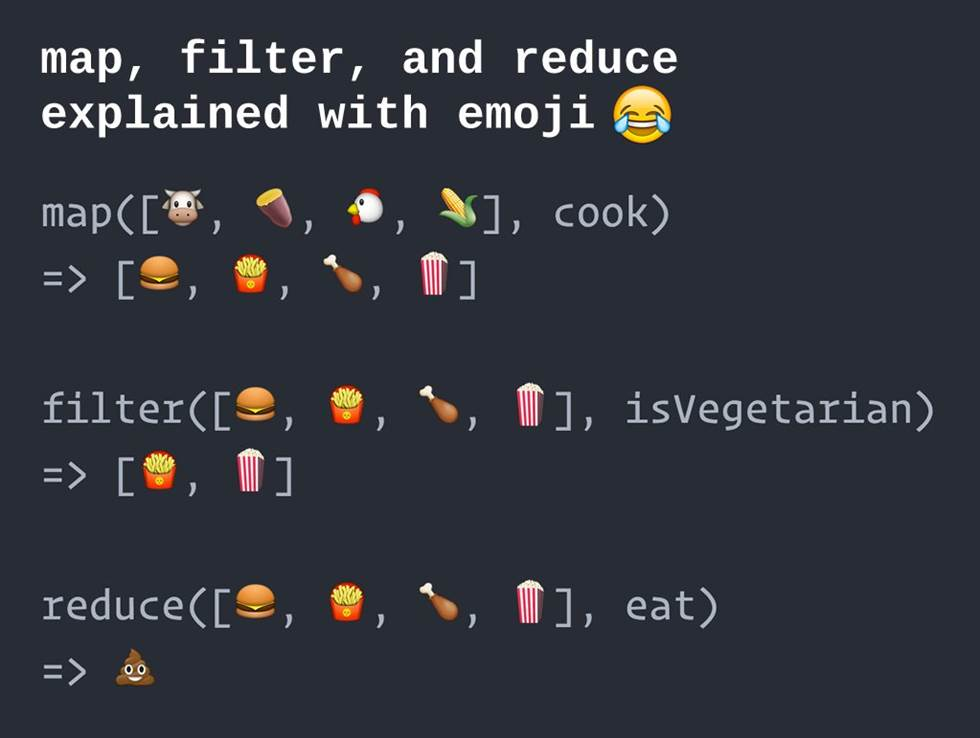##Modernes C++ Mentoring

Be part of my mentoring programs:

• "Fundamentals for C++ Professionals" (open)
• "Design Patterns and Architectural Patterns with C++" (open)
• "C++20: Get the Details" (reopens December 2023)
• Do you want to stay informed about my mentoring programs: Subscribe via E-Mail.

#### The name variations

The statement that each programming language supporting programming in a functional style has to support the three functions map, filter, and reduce, has few restrictions. The names of the three functions have variations in the different programming languages. You can see in the table the names of the Haskell functions in comparison with their names in C++ and Python.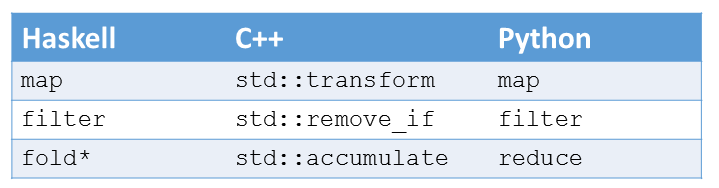I want to stress two points. Firstly, Haskell has four variations of the fold. The variations are due to the fact if the binary operation starts at the beginning or at the end of the list and if the binary operation has an initial value. Secondly, string concatenation of map and reduce (fold) gives MapReduce. This is no accident. The Google framework is based on a map and a reduce phase and is therefore based on the ideas of the functions map and reduce (fold).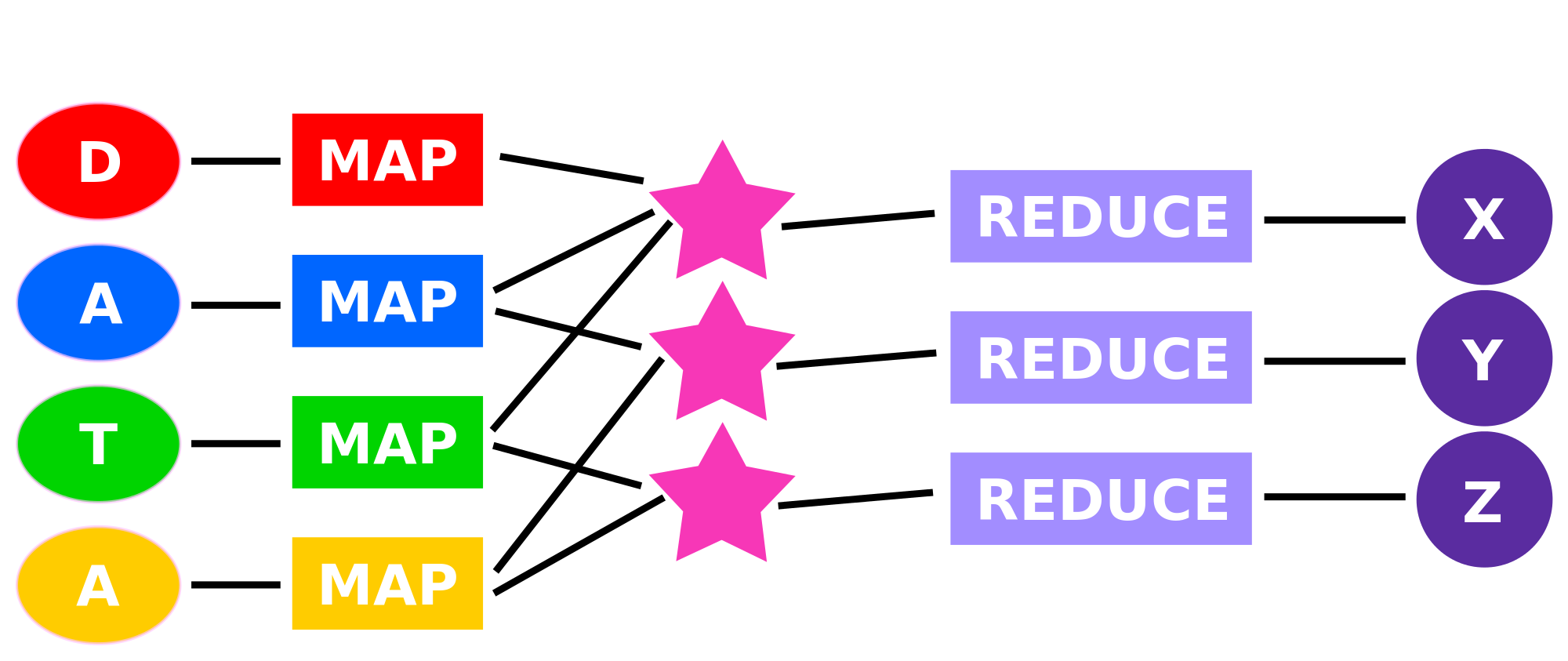The easiest way to understand the functions is to use them. As input, I choose a list (vec) with the integers from 0 to 9 and a list (str) with the words “Programming”,”in”,”a”,”functional”,”style.” In the case of C++, the list is a std::vector.

```// Haskell
vec = [1..9]
str = ["Programming","in","a","functional","style."]

// Python
vec=range(1, 10)
str=["Programming", "in", "a", "functional", "style."]

// C++
std::vector<int> vec{1, 2, 3, 4, 5, 6, 7, 8, 9}
std::vector<string> str{"Programming", "in", "a", "functional", "style."}
```

For simplicity reasons, I will directly display the results in the list syntax of Haskell.

#### map

map applies a callable to each element of a list. A callable is all that behaves like a function. A callable can be, in C++, a function, a function object, or a lambda function. The best fit for higher-order functions is often a lambda function. That is for two reasons. On the one hand, you can concisely express your intent, and the code is easier to understand. On the other hand, the lambda function defines its functionality precisely where it is used. Because of that, the compiler gets maximum insight into the source code and has the maximum potential to optimize. That is the reason that you will often get more performant executables with a lambda function

```// Haskell
map(\a -> a * a) vec
map(\a -> length a) str

// Python
map(lambda x :x*x , vec)
map(lambda x : len(x), str)

// C++
std::transform(vec.begin(), vec.end(), vec.begin(),
[](int i){ return i*i;} );
std::transform(str.begin(), str.end(), std::back_inserter(vec2),
[](std::string s){ return s.length ();} );

// [1,4,9,16,25,36,49,64,81]
// [11,2,1,10,6]
```

Of course, the syntax of lambda functions in Haskell, Python, and C++ is different. Therefore, a lambda function will be introduced in Haskell by a slash \a → a*a, but will be introduced in Python by the lambda: lambda x: x*x. In C++, you have to use square brackets: [](int i){ return i*i; }. These are only syntactical differences. More interesting is that you invoke functions in Haskell without braces and that Haskell and Python generate a list, while you can modify in C++ the existing std::vector or fill a new one.

#### filter

filter keeps only these elements in the list that satisfy the predicate. A predicate is a callable that returns a boolean. Here is an example.

```// Haskell
filter (\x-> x <3 || x> 8) vec
filter (\x -> isupper (head x)) sts

// Python
filter(lambda x: x<3 or x>8 , vec)
filter(lambda x: x.isupper(), str)

// C++
auto it = std::remove_if(vec.begin(), vec.end (),
[](int i){return ((i <3) or (i> 8))!});
auto it2 = std::remove_if(str.begin(),  str.end (),
[](std::string s) {return !(std::isupper (s));});

// [1,2,9]
// ["Programming"]
```

The function composition isUpper(head x) checks for each word if it starts (head x) with a capital letter  (isUpper) ist.

Two quick remarks to std::remove_if. std::remove_if removes no element but returns the list’s new logical end. Afterward, you have to apply the erase-remove idiom. The logic of std::remove_if is the other way around. It will remove the elements satisfying the condition. Therefore, I have to negate the condition.

#### fold

fold is the most powerful of the three higher-order functions. You can implement map and filter by using fold. The code snippet shows the calculation of the faculty of 9 and string concatenation in Haskell, Python, and C++.

```// Haskell
foldl (\a b -> a * b) 1 vec
foldl (\a b -> a ++ ":" ++ b ) "" str

//Python
reduce(lambda a , b: a * b, vec, 1)
reduce(lambda a, b: a + ":" + b, str, "")

// C++
std::accumulate(vec.begin(), vec.end(), 1,
[](int a, int b){ return a*b; });
std::accumulate(str.begin(), str.end(), string(""),
[](std::string a,std::string b){ return a+":"+b; });

// 362800
// ":Programming:in:a:functional:style."
```

foldl needs as the Python pendant reduce and the C++ pendant std::accumulate an initial value. This is in the case of the faculty the 1; this is in the case of the string concatenation the empty string “”.  Haskell requires two ++ symbols for adding two strings; Python and C++ only one.

The strategy of std::accumulate is a little bit difficult to get. Therefore, I have a graphic visualization of the processing.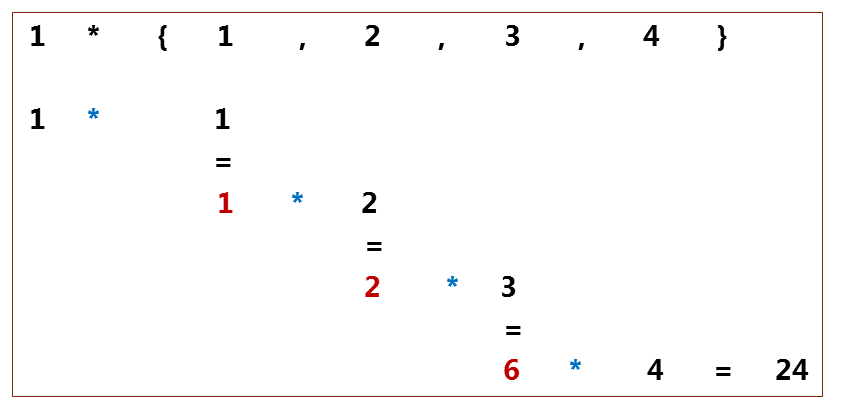In the first iteration, the initial value 1 together with the first value of the input sequence is used as the arguments for the lambda function: 1*1= 1. The result 1 is the first argument for the lambda function in the second iteration: 1*2= 2. The third iteration has the previous result 2 as the first argument: 2*3= 6. The last iteration returns the final result 24.

## What’s next?

Pure functional languages such as Haskell have the characteristic that their data is immutable. Therefore, Haskell can not have a for, while, or until loop. In the next post, I will write about the consequences of Haskell.

Thanks a lot to my Patreon Supporters: Matt Braun, Roman Postanciuc, Tobias Zindl, G Prvulovic, Reinhold Dröge, Abernitzke, Frank Grimm, Sakib, Broeserl, António Pina, Sergey Agafyin, Андрей Бурмистров, Jake, GS, Lawton Shoemake, Jozo Leko, John Breland, Venkat Nandam, Jose Francisco, Douglas Tinkham, Kuchlong Kuchlong, Robert Blanch, Truels Wissneth, Kris Kafka, Mario Luoni, Friedrich Huber, lennonli, Pramod Tikare Muralidhara, Peter Ware, Daniel Hufschläger, Alessandro Pezzato, Bob Perry, Satish Vangipuram, Andi Ireland, Richard Ohnemus, Michael Dunsky, Leo Goodstadt, John Wiederhirn, Yacob Cohen-Arazi, Florian Tischler, Robin Furness, Michael Young, Holger Detering, Bernd Mühlhaus, Matthieu Bolt, Stephen Kelley, Kyle Dean, Tusar Palauri, Dmitry Farberov, Juan Dent, George Liao, Daniel Ceperley, Jon T Hess, Stephen Totten, Wolfgang Fütterer, Matthias Grün, Phillip Diekmann, Ben Atakora, Ann Shatoff, Rob North, Bhavith C Achar, and Marco Parri Empoli.

Thanks, in particular, to Jon Hess, Lakshman, Christian Wittenhorst, Sherhy Pyton, Dendi Suhubdy, Sudhakar Belagurusamy, Richard Sargeant, Rusty Fleming, John Nebel, Mipko, Alicja Kaminska, Slavko Radman, and David Poole.

 My special thanks to EmbarcaderoMy special thanks to PVS-StudioMy special thanks to Tipi.buildMy special thanks to Take Up Code## Seminars

I’m happy to give online seminars or face-to-face seminars worldwide. Please call me if you have any questions.

### Standard Seminars (English/German)

Here is a compilation of my standard seminars. These seminars are only meant to give you a first orientation.

• C++ – The Core Language
• C++ – The Standard Library
• C++ – Compact
• C++11 and C++14
• Concurrency with Modern C++
• Design Pattern and Architectural Pattern with C++
• Embedded Programming with Modern C++
• Generic Programming (Templates) with C++

#### New

• Clean Code with Modern C++
• C++20

### Contact Me

Modernes C++ Mentoring,0 replies